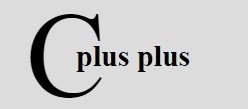# C++ Preprocessor

« Previous Tutorial Next Tutorial »

Preprocessors in C++, are basically the directives, instructs the C++ compiler to pre-process the information before the actual program compilation.

## #define Preprocessor in C++

Macros are built on the #define preprocessor. Normally a #define would look like :

`#define PI 3.142`

But, a macro would look like this (also called as function macro).

`#define SQUARE(x) x∗x`

The main difference is that the first example is a symbolic constant and the second is an expression. If the macro above was used in some code it may look like this :

```/* C++ Preprocessor - Example Program */

#include<iostream.h>
#include<conio.h>

#define SQUARE(x) x*x

void main()
{
clrscr();
int val;
cout<<"Enter a number: ";
cin>>val;
cout<<"Square = "<<SQUARE(val);
getch();
}```

After processing the code would become :

```void main()
{
clrscr();
int val;
cout<<"Enter a number: ";
cin>>val;
cout<<"Square = "<<val*val;
getch();
}```

Here is the sample run of this C++ program:

The text replacement for a macro, is known as macro expansion.

A few things that you must know about macros are :

• A macro without argument is treated like a symbolic constant.
• A macro with arguments has its arguments substituted for replacement text, when the macro is expanded.
• A macro substitutes text only; it does not check for data types.

While defining macros, make sure to use parenthesis, as it ensures the correct result. For instance, if you have defined a macro like :

`#define CIRCLE_AREA(x) PI∗x∗x`

and you are using it as :

`area = CIRCLE_AREA(c + 2) ;`

then it would be expanded as :

`area = 3.14159 ∗ c + 2 ∗ c + 2 ;`

See, would it yield the correct result? To obtain the correct result, you should define this macro as :

`#define CIRCLE_AREA((x)) PI∗(x)∗(x)`

would cause

`rectArea = RECTANGLE_AREA(x + 4, y +7) ;`

be expanded as

`rectArea = ((x+4) ∗ (y+7)) ;`

Here is an example, illustrating the #define preprocessor in C++

```/* C++ Preprocessor - Example Program */

#include<iostream.h>
#include<conio.h>
#define PI 3.14
void main()
{
clrscr();
cout<<"Value of PI is "<<PI;
getch();
}```

Here is the sample output of the above C++ program:

Let's take one more example, demonstrating preprocessor in C++

```/* C++ Preprocessor - Example Program */

#include<iostream.h>
#include<conio.h>
#define PI 3.14
void main()
{
clrscr();
cout<<"Enter radius of the circle: ";
getch();
}```

Here is the sample run of the above C++ program:

For more detail about #define, refer C++ #define

## Function Macros in C++

Here is an example program demonstrating the function macros (preprocessor) in C++

```/* C++ Preprocessors - Example Program */

#include<iostream.h>
#include<conio.h>
#define MIN(num1, num2) (((num1)<(num2))?num1:num2)
void main()
{
clrscr();
int a, b;
cout<<"Enter any two number: ";
cin>>a>>b;
cout<<"Minimum of the two number is "<<MIN(a, b);
getch();
}```

Below is the sample run of the above C++ program:

Here is one more example program, also demonstrating preprocessor in C++

```/* C++ Preprocessors - Example Program */

#include<iostream.h>
#include<conio.h>
#define MAX(num1, num2) (((num1)>(num2))?num1:num2)
void main()
{
clrscr();
int a, b;
cout<<"Enter any two number: ";
cin>>a>>b;
cout<<"Maximum of the two number = "<<MAX(a, b);
getch();
}```

Below is the sample run of this C++ program:

## Conditional Compilation in C++

Here is an example program, demonstrating the conditional compilation in C++:

```/* C++ Preprocessor - Example Program */

#include<iostream.h>
#include<conio.h>
#define DEBUG
#define MIN(num1, num2) (((num1)<(num2))?num1:num2)
void main()
{
clrscr();
int a, b;
cout<<"Enter two number: ";
cin>>a>>b;
#ifdef DEBUG
cerr<<"Trace: inside the main() function\n";
#endif
#if 0
cout<<MKSTR(HELLO C++)<<endl;
#endif
cout<<"The minimum = "<<MIN(a, b)<<"\n";
#ifdef DEBUG
cerr<<"Trace: coming out of the main() function\n";
#endif
getch();
}```

Here is the sample run of the above C++ program:

Below is another example program, also demonstrating the conditional compilation in C++

```/* C++ Preprocessor - Example Program */

#include<iostream.h>
#include<conio.h>
#define DEBUG
#define MAX(num1, num2) (((num1)>(num2))?num1:num2)
void main()
{
clrscr();
int a, b;
cout<<"Enter two number: ";
cin>>a>>b;
#ifdef DEBUG
cerr<<"Trace: inside the main() function\n";
#endif
#if 0
// this is commented part
cout<<MKSTR(HELLO C++)<<endl;
#endif
cout<<"The maximum of the two number = "<<MAX(a, b)<<"\n";
#ifdef DEBUG
cerr<<"Trace: coming out of the main() function\n";
#endif
getch();
}```

Following is the sample run of the above C++ program:

## # and ## Operators in C++

The # operator causes a replacement-text token to be converted to a string surrounded by the quotes. Here is an example demonstrating this.

```/* C++ Preprocessor - Example Program */

#include<iostream.h>
#include<conio.h>
#define MKSTR(z) #z
void main()
{
clrscr();
cout<<MKSTR(HELLO C++)<<endl;
getch();
}```

Following is the sample output of the above C++ program:

Here the line:

`cout<<MKSTR(HELLO C++)<<endl;`

becomes

`cout<<"HELLO C++"<<endl;`

The ## operator is used to concatenate two tokens. Below is an example program demonstrating this concept:

```/* C++ Preprocessor - Example Program */

#include<iostream.h>
#include<conio.h>
#define concat(i, j) i##j
void main()
{
clrscr();
int ab=100;
cout<<concat(a, b);
getch();
}```

Here is the sample output of this C++ program:

Here the line

`cout<<concat(a, b);`

becomes

`cout<<ab;`

C++ Online Test

« Previous Tutorial Next Tutorial »